# Bar Plot in PythonA bar plot shows catergorical data as rectangular bars with the height of bars proportional to the value they represent. It is often used to compare between values of different categories in the data.

## Content

1. What is a barplot?
2. Simple bar plot using matplotlib
3. Horizontal barplot
4. Changing color of a barplot
5. Grouped and Stacked Barplots
6. Grouped barplot
7. Stacked barplot
8. List of available palettes

Get FREE 30 DAY ACCESS  to Structured learning path for ML in Python (Begnner to Pro) –  Start as an absolute beginner and graduate over 15 courses to solve Industrial Projects like a real Data Scientist. Includes query support over chat.

## What is a barplot?

A bar plot shows catergorical data as rectangular bars with heights proportional to the value they represent. It is often used to compare between values of different categories in the data.

What is categorical data?

A categorical data is nothing but a grouping of data into different logical groups, for example, data on the height of persons being grouped as ‘Tall’, ‘Medium’, ‘Short’ etc.

To make a bar plot, you need to calculate the count of each category.

First you need to install all the required libraries which we will be using. I also changed the default figsize and dpi (dots per inch) parameters by using plt.rcParams.update() function.

import numpy as np
import pandas as pd
import matplotlib.pyplot as plt
%matplotlib inline
plt.rcParams.update({'figure.figsize':(7,5), 'figure.dpi':100})


Lets create a dataset containing 10 discrete categories and assign values to each catergory.

To create a random array, use np.random.randn() function with lower limit, upper limit and size of the array as arguments.

# Create Data
x=['A','B','C','D','E','F','G','H','I','J']
y = np.random.randint(low=0, high=100, size=10)
y

array([47, 23, 27,  0, 82,  7, 46, 92, 36, 76])


You can see that y contains an array of randomly assigned values

## Simple bar plot using matplotlib

For plotting a barplot in matplotlib, use plt.bar() function passing 2 arguments – ( x_value , y_value)

# Simple Bar Plot
plt.bar(x,y)
plt.xlabel('Categories')
plt.ylabel("Values")
plt.title('Categories Bar Plot')
plt.show()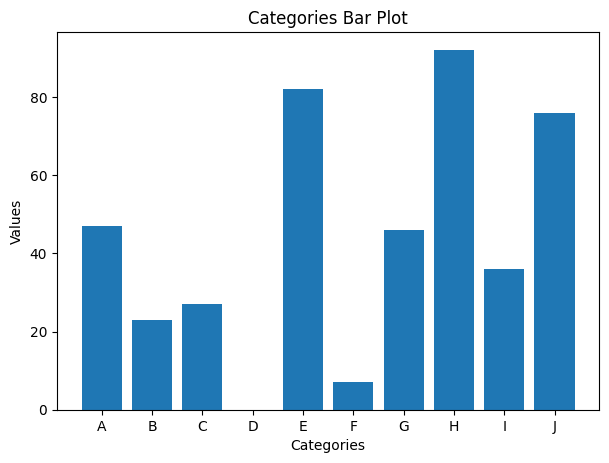In the above barplot we can visualize the array we just created using random() function.

## Horizontal barplot

You can also visualize the same graph horizontally using the barh() function with the same values as arguments.

# Horizontal Bar plot
plt.barh(x,y)
plt.xlabel("Values")
plt.ylabel('Categories')
plt.title('Horizontal Bar Plot')
plt.show()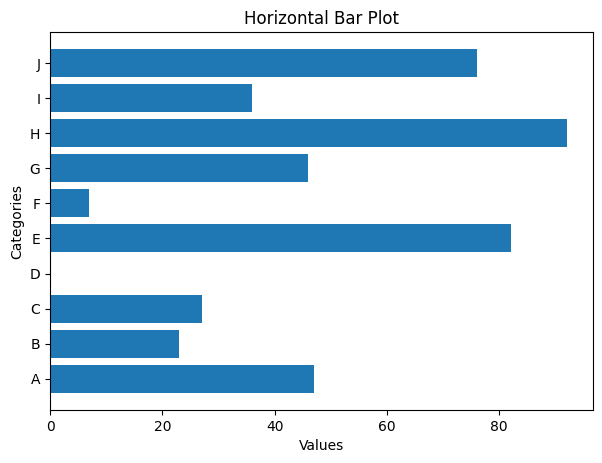Get FREE 30 DAY ACCESS  to Structured learning path for ML in Python (Beginner to Pro) –  Start as an absolute beginner and graduate over 15 courses to solve Industrial Projects like a real Data Scientist. Includes query support over chat.

## Changing color of a barplot

You can also change the color of the bar by using the [color= ‘ ‘] command in the plt.bar() fucntion.

# Change color of each bar
plt.bar(x,y, color=['firebrick', 'green', 'blue', 'black', 'red',
'purple', 'seagreen', 'skyblue', 'black', 'tomato'])
plt.xlabel('Categories')
plt.ylabel("Values")
plt.title('Barplot with colored bars')
plt.show()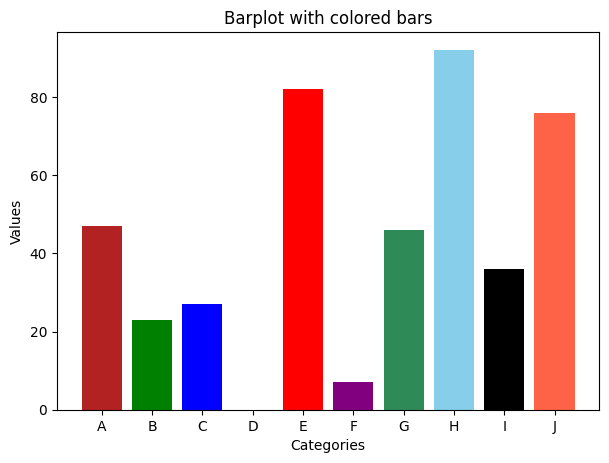## Grouped and Stacked Barplots

There are 2 types of barplots – Grouped and stacked barplots.

Let’s look into this with an example of the famous titanic dataset.

This dataset contains the data of whether the person has survived or not during the sink of titanic and different details of the person.

## Grouped barplot

In a grouped bar chart, for each categorical group there are two or more bars.

# Import data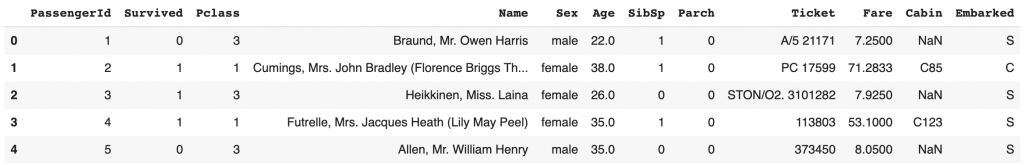If you want to plot Grouped barplots then use seaborn package using hue='groupColumnName' which will contain the category which you are grouping into x.

So basically you are splitting the x('Sex') category further into categories 'Pclass' .

This is called as grouped barplot.

# Grouped bar plot with seaborn
import seaborn as sns
sns.barplot(y='Survived',x='Sex',hue='Pclass',data=df);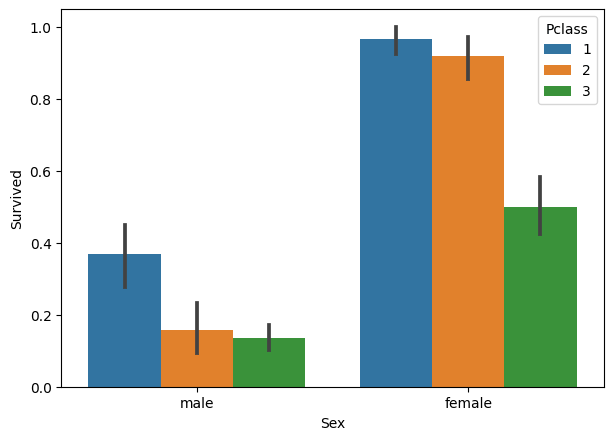Let’s see another example.

# Another example
sns.barplot(y='Survived',x='Sex',hue='SibSp',data=df);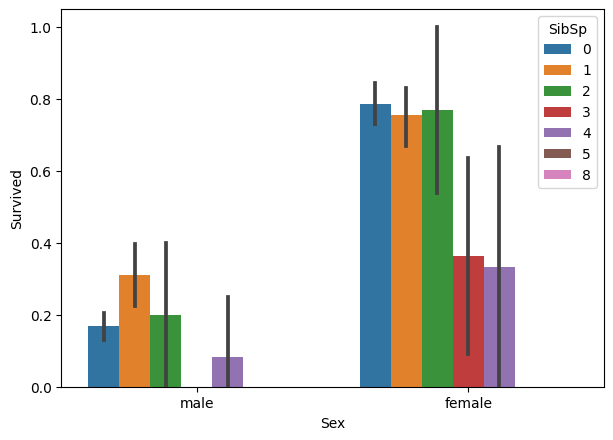You can also see the error bar for each category.

Error bars are graphical representations of the variability of data and used on graphs to indicate the error or uncertainty in a reported measurement.

Error bars often represent one standard deviation of uncertainty, one standard error, or a particular confidence interval (e.g., a 95% interval).

## Stacked barplot

The stacked bar chart stacks bars that represent different groups on top of each other.

This can be done in pandas library by using stacked='True' command in df.plot() function.

# Stacked barplot with pandas
survived = df.loc[df['Survived']==1, :]['Pclass'].value_counts()
died     = df.loc[df['Survived']==0, :]['Pclass'].value_counts()
df_plot  = pd.DataFrame([survived,died])
df_plot.index=['survived','died']

# Plot
df_plot.plot(kind='bar',stacked=True, title='Stacked Bar plot');The above graph is categorized based on whether the passenger survived or not and also stacked based on the class in which the passenger is traveling.

Let’s draw with different palette.

# Stacked barplot with pandas with differnt palette
survived = df.loc[df['Survived']==1, :]['Sex'].value_counts()
died     = df.loc[df['Survived']==0, :]['Sex'].value_counts()
df_plot  = pd.DataFrame([survived,died])
df_plot.index=['survived','died']

# Bar plot
df_plot.plot(kind='bar',stacked=True, colormap='Spectral', title='Stacked Bar plot with Spectral Palette');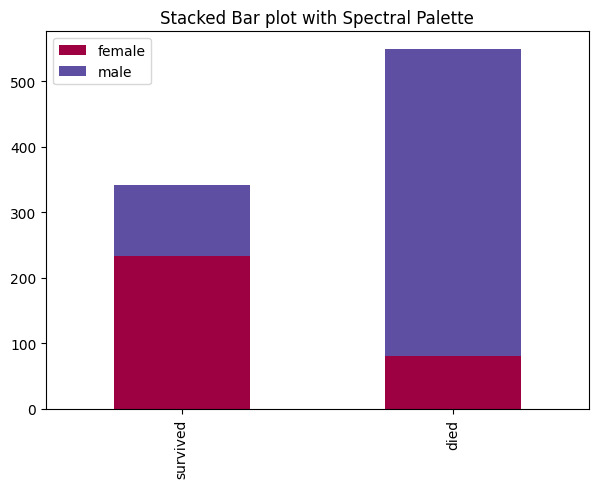The above graph is categorized based on whether the passenger survived or not and also stacked based on the number of siblings of each passenger.

The difference between the 2 barplots is that Grouped bar graphs usually present the information in the same order in each grouping whereas Stacked bar graphs present the information in the same sequence on each bar.

## List of available palettes

# List of available Palettes
import matplotlib.cm as cm
maps=[m for m in cm.datad if not m.endswith("_r")]
print(maps)

['Blues', 'BrBG', 'BuGn', 'BuPu', 'CMRmap', 'GnBu', 'Greens', 'Greys', 'OrRd', 'Oranges',
'PRGn', 'PiYG', 'PuBu', 'PuBuGn', 'PuOr', 'PuRd', 'Purples', 'RdBu', 'RdGy', 'RdPu', 'RdYlBu', 'RdYlGn',
'Reds', 'Spectral', 'Wistia', 'YlGn', 'YlGnBu', 'YlOrBr', 'YlOrRd', 'afmhot', 'autumn', 'binary', 'bone',
'brg', 'bwr', 'cool', 'coolwarm', 'copper', 'cubehelix', 'flag', 'gist_earth', 'gist_gray', 'gist_heat',
'gist_ncar', 'gist_rainbow', 'gist_stern', 'gist_yarg', 'gnuplot', 'gnuplot2', 'gray', 'hot', 'hsv', 'jet',
'nipy_spectral', 'ocean', 'pink', 'prism', 'rainbow', 'seismic', 'spring', 'summer', 'terrain', 'winter',
'Accent', 'Dark2', 'Paired', 'Pastel1', 'Pastel2', 'Set1', 'Set2', 'Set3', 'tab10', 'tab20', 'tab20b',
'tab20c']

## Recommended Posts

Get FREE 30 DAY ACCESS  to Structured learning path for ML in Python (Beginner to Pro) –  Start as an absolute beginner and graduate over 15 courses to solve Industrial Projects like a real Data Scientist. Includes query support over chat.Reference project template for all your Data Science projects. Learn  how to load the data, get an overview of the data. Perform EDA, prepare data, build models & improve model performance.

## Similar Articles

### Logistic Regression – A Complete Tutorial With Examples in RReference project template for all your Data Science projects. Learn  how to load the data, get an overview of the data. Perform EDA, prepare data, build models & improve model performance.Course Preview

## Machine Learning A-Z™: Hands-On Python & R In Data Science

### Free Sample Videos:#### Machine Learning A-Z™: Hands-On Python & R In Data Science#### Machine Learning A-Z™: Hands-On Python & R In Data Science#### Machine Learning A-Z™: Hands-On Python & R In Data Science#### Machine Learning A-Z™: Hands-On Python & R In Data Science